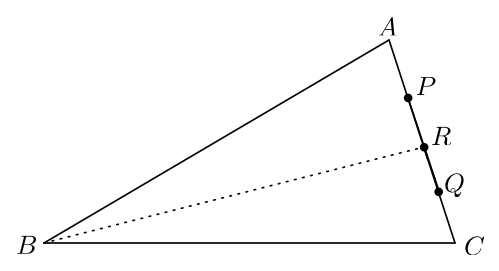# The Internal Angle Bisector

Geometry Level 3In $\triangle ABC$, $\angle ABC = 30°.$ Points $P$ and $Q$ are chosen on $\overline{AC}$ such that $AP+BC= AB+CQ$. The internal angle bisector of $\angle ABC$ intersects $\overline{AC}$ at $R$.

Given that $R$ is the midpoint of $PQ$, find $\angle BAC$ (in degrees).

×